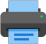Lista de exercícios do ensino médio para impressãoSelect all.
print selected exercises
(FESP - 1991) An equilateral triangle ABC is inscriibed in a circle whose radius is 6 cm length. The triangle is intercepted by a diameter MN of the circle, forming a trapezoid, as shown in the picture below. We can say that the quotient of the triangle ABC area divided by the trapezoid APQC area is:
a)
$\,\dfrac{5}{4}\,$
b)
$\,\dfrac{9}{5}\,$
c)
$\,\dfrac{9}{8}\,$
d)
$\,\dfrac{9}{4}\,$
e)
$\,\dfrac{8}{5}\,$